# How can Tensorflow be used to load the flower dataset and work with it?

• Last Updated : 27 Jun, 2022

Tensorflow flower dataset is a large dataset of images of flowers. In this article, we are going to see, how we can use Tensorflow to load the flower dataset and work with it.

Let us start by importing the necessary libraries. Here we are going to use the tensorflow_dataset library to load the dataset. It is a library of public datasets ready to use with TensorFlow. If you don’t have any of the libraries mentioned below, you can install them using the pip command, for example, to install tensorflow_datasets library you need to write the following command:

`pip install tensorflow-datasets`

## Python3

 `# Importing libraries``import` `tensorflow as tf``import` `numpy as np``import` `pandas as pd``import` `tensorflow_datasets as tfds`

To import the flower dataset, we are going to use the tfds.load() method. It is used to load the named dataset, which is provided using the name argument, into a tf.data.Dataset. The name for the flower dataset is tf_flowers. In the method, we also split the dataset using the split argument with training_set taking 70% of the dataset and the rest going to test_set.

## Python3

 `(training_set, test_set), info ``=` `tfds.load(``    ``'tf_flowers'``,``    ``split``=``[``'train[:70%]'``, ``'train[70%:]'``],``    ``with_info``=``True``,``    ``as_supervised``=``True``,``)`

If we print the information provided for the dataset by Tensorflow using the print command, we will get the following output:

## Python3

 `print``(info)`

Output: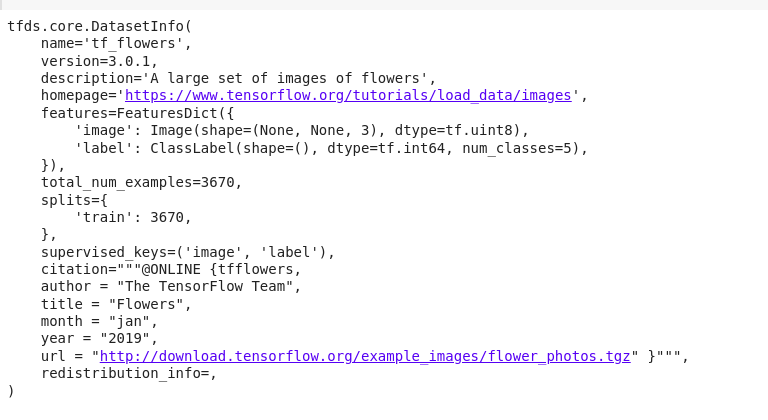The flower dataset contains 3670 flower images, which is distributed in the following fashion in training_set and test_set.

## Python3

 `print``(``"Training Set Size: %d"` `%` `training_set.cardinality().numpy())``print``(``"Test Set Size: %d"` `%` `test_set.cardinality().numpy())`

Output: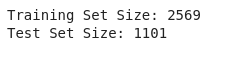The flower dataset consists of images of 5 different kinds of flowers.

## Python3

 `num_classes ``=` `info.features[``'label'``].num_classes``print``(``"Number of Classes: %d"` `%` `num_classes)`

Output: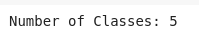Let us now visualize some of the images in the dataset. The following code displays the first 5 images in the dataset.

## Python3

 `import` `matplotlib.pyplot as plt`` ` `ctr ``=` `0``plt.rcParams[``"figure.figsize"``] ``=` `[``30``, ``15``]``plt.rcParams[``"figure.autolayout"``] ``=` `True`` ` `for` `image, label ``in` `training_set:``    ``image ``=` `image.numpy()``    ``plt.subplot(``1``, ``5``, ctr``+``1``)``    ``plt.title(``'Label {}'``.``format``(label))``    ``plt.imshow(image, cmap``=``plt.cm.binary)``    ``ctr ``+``=` `1``    ``if` `ctr ``=``=` `5``:``        ``break`` ` `plt.show()`

Output: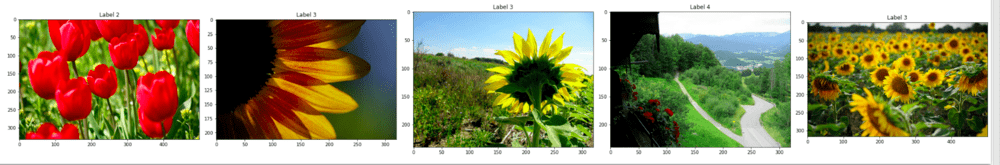If you might observe carefully, the different images don’t have the same size rather they have different sizes. We can verify this by printing the sizes of the images we visualized just now. The following code accomplishes the goal:

## Python3

 `for` `i, example ``in` `enumerate``(training_set.take(``5``)):``    ``shape ``=` `example[``0``].shape``    ``print``(``"Image %d -> shape: (%d, %d) label: %d"` `%``          ``(i, shape[``0``], shape[``1``], example[``1``]))`

Output: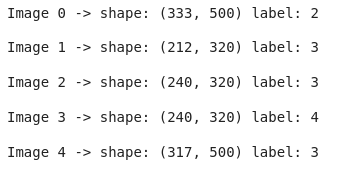As you might observe the shapes of the various images are different.

However, for the purposes of feeding this dataset into a machine learning model, we will need to have all images be of the same size. For this, we will preprocess the images a little. Namely, we will resize all the images to a fixed size which is 224 in this case, and normalize the images so that the value of each pixel comes in the range 0 to 1. The following piece of code serves the desired purpose.

## Python3

 `IMG_SIZE ``=` `224`` ` `def` `format_image(image, label):`` ` `    ``image ``=` `tf.image.resize(image, (IMG_SIZE, IMG_SIZE))``     ` `    ``# Normalisation``    ``image ``=` `image``/``255.0``    ``return` `image, label`` ` `batch_size ``=` `32``training_set ``=` `training_set.shuffle(``300``).``map``(``    ``format_image).batch(batch_size).prefetch(``1``)``test_set ``=` `test_set.``map``(format_image).batch(batch_size).prefetch(``1``)`

Printing both the datasets reveals that rightfully each image in the dataset has now been resized, with each image being of size (224,224,3).

## Python3

 `print``(training_set)``print``(test_set)`

Output: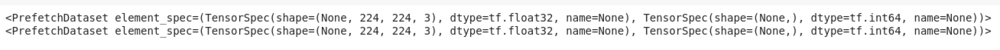Now you can feed this dataset to any appropriate machine learning model.

For the purposes of demonstration, we will use a modified version of MobileNet to train on this dataset. The following is the piece of code that describes the model, optimizer, loss function, and metric used while training the model.

## Python3

 `def` `getModel(image_shape):``    ``mobileNet ``=` `tf.keras.applications.mobilenet.MobileNet(image_shape)``    ``X ``=` `mobileNet.layers[``-``2``].output``    ``X_output ``=` `tf.keras.layers.Dense(``1``,``                                     ``activation``=``'relu'``)(X)``    ``model ``=` `tf.keras.models.Model(inputs``=``mobileNet.``input``,``                                  ``outputs``=``X_output)``    ``return` `model`` ` `model ``=` `getModel((IMG_SIZE, IMG_SIZE, ``3``))`` ` `optimizer ``=` `tf.keras.optimizers.Adam()``loss ``=` `'mean_squared_error'``model.``compile``(optimizer``=``optimizer, ``              ``loss``=``loss,``              ``metrics``=``'accuracy'``)`` ` `epochs ``=` `5``model.fit(training_set, epochs``=``epochs, ``          ``validation_data``=``test_set)`

Output: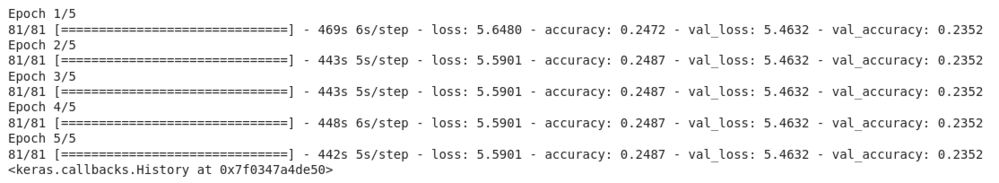The model performs measly on the dataset right now. You can train the model for a longer number of epochs as well as use one-hot encoding for the output variable to increase the accuracy.

My Personal Notes arrow_drop_up